# How to count distinct and unique values in Excel

In this tutorial, you will learn how to count unique values in Excel with formulas, and how to get an automatic count of distinct values in a pivot table. We will also discuss a number of formula examples for counting unique names, texts, numbers, cased-sensitive unique values, and more.

When working with a large dataset in Excel, you may often need to know how many duplicate and unique values are there. And sometimes, you may want to count only the distinct (different) values.

If you have been visiting this blog on a regular basic, you already know the Excel formula to count duplicates. And today, we are going to explore different ways to count unique values in Excel. But for the sake of clarity, let's define the terms first.

• Unique values - these are the values that appear in the list only once.
• Distinct values - these are all different values in the list, i.e. unique values plus 1st occurrences of duplicate values.

The following screenshot demonstrates the difference: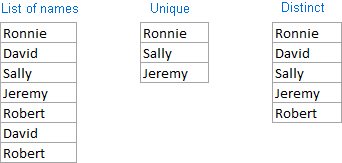And now, let's see how you can count unique and distinct values in Excel using formulas and PivotTable features.

## How to count unique values in Excel

Here's a common task that all Excel users have to perform once in a while.  You have a list of data and you need to find out the number of unique values in that list. How do you do that? Easier than you may think :) Below you will find a few formulas to count unique values of different types.

### Count unique values in a column

Supposing you have a column of names in your Excel worksheet, and you need to count unique names in that column. The solution is to use the SUM function in combination with IF and COUNTIF:

=SUM(IF(COUNTIF(range, range)=1,1,0))

Note. This is an array formula, so be sure to press Ctrl + Shift + Enter to complete it. Once you do this, Excel will automatically enclose the formula in {curly braces} like in the screenshot below. In no case should you type the curly braces manually, that won't work.

In this example, we are counting unique names in range A2:A10, so our formula takes the following shape:

`=SUM(IF(COUNTIF(A2:A10,A2:A10)=1,1,0))`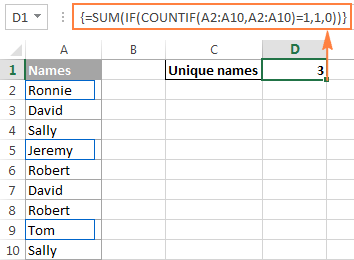Further on in this tutorial, we are going to discuss a handful of other formulas to count unique values of different types. And because all those formulas are variations of the basic Excel unique values formula, it makes sense to break down the above formula, so you can fully understand how it works and tweak it for your data. If someone is not interested in technicalities, you can skip right to the next formula example.

#### How the Excel count unique values formula works

As you see, 3 different functions are used in our unique values formula - SUM, IF and COUNTIF. Looking from the inside out, here's what each function does:

• The COUNTIF function counts how many times each individual value appears in the specified range.

In this example, `COUNTIF(A2:A10,A2:A10)` returns the array `{1;2;2;1;2;2;2;1;2}`.

• The IF function evaluates each value in the array returned by COUNTIF, keeps all 1's (unique values), and replaces all other values with zeros.

So, the function `IF(COUNTIF(A2:A10,A2:A10)=1,1,0)` becomes `IF(1;2;2;1;2;2;2;1;2) = 1,1,0,` which turns into the array `{1;0;0;1;0;0;0;1;0}` where 1 is a unique value and 0 is a duplicate value.

• Finally, the SUM function adds up the values in the array returned by IF and outputs the total number of unique values, which is exactly what we wanted.
Tip. To see what a specific part of your Excel unique values formula evaluates to, select that part in the formula bar and press the F9 key.

### Count unique text values in Excel

If your Excel list contains both numerical and text values, and you want to count only unique text values, add the ISTEXT function to the array formula discussed above:

`=SUM(IF(ISTEXT(A2:A10)*COUNTIF(A2:A10,A2:A10)=1,1,0))`

As you know, the Excel ISTEXT function returns TRUE if an evaluated value is text, FALSE otherwise. Since the asterisk (*) works as the AND operator in array formulas, the IF function returns 1 only if a value is both text and unique, 0 otherwise. And after the SUM function adds up all 1's, you will get a count of unique text values in the specified range.

Don't forget to press Ctrl + Shift + Enter to correctly enter the array formula, and you will get a result similar to this: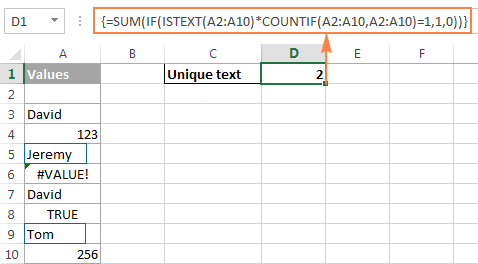As you can see in the screenshot above, the formula returns the total number of unique text values, excluding blank cells, numbers, logical values of TRUE and FALSE, and errors.

### Count unique numeric values in Excel

To count unique numbers in a list of data, utilize an array formula like we've just used for counting unique text values, with the only difference that you embed ISNUMBER instead of ISTEXT in your unique values formula:

`=SUM(IF(ISNUMBER(A2:A10)*COUNTIF(A2:A10,A2:A10)=1,1,0))`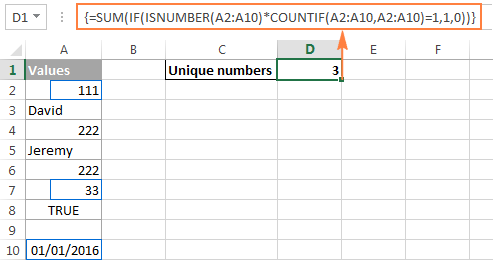Note. Since Microsoft Excel stores dates and times as serial numbers, they are also counted.

### Count case-sensitive unique values in Excel

If your table contains case-sensitive data, the easiest way to count unique values would be creating a helper column with the following array formula to identify duplicate and unique items:

`=IF(SUM((--EXACT(\$A\$2:\$A\$10,A2)))=1,"Unique","Dupe")`

And then, use a simple COUNTIF function to count unique values:

`=COUNTIF(B2:B10, "unique")`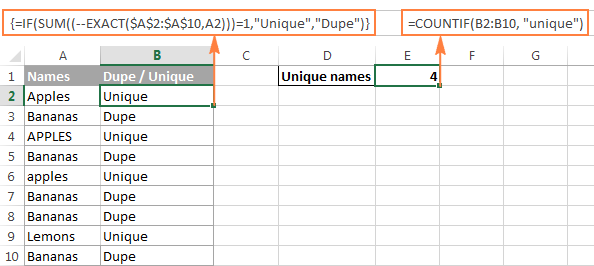## Count distinct values in Excel (unique and 1st duplicate occurrences)

To get a count of distinct values in a list, use the following formula:

=SUM(1/COUNTIF(range, range))

Remember, it's an array formula, and therefore you should press the Ctrl + Shift + Enter shortcut instead of the usual Enter keystroke.

Alternatively, you can use the SUMPRODUCT function and complete the formula in the usual way by pressing the Enter key:

=SUMPRODUCT(1/COUNTIF(range, range))

For example, to count the distinct values in range A2:A10, you can go with either:

`=SUM(1/COUNTIF(A2:A10,A2:A10))`

Or

`=SUMPRODUCT(1/COUNTIF(A2:A10,A2:A10))`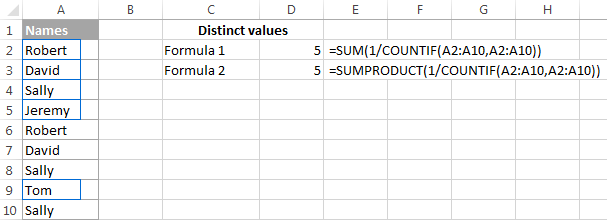#### How the Excel distinct formula works

As you already know, we use the COUNTIF function to find out how many times each individual value appears in the specified range. In the above example, the result of the COUNTIF function is the following array: `{2;2;3;1;2;2;3;1;3}`.

After that, a number of division operations are performed, where each value of the array is used as a divisor with 1 as the dividend. This turns all duplicates values into fractional numbers corresponding to the number of duplicate occurrences. For example, if a value appears 2 times in the list, it generates 2 items in the array with a value of 0.5 (1/2=0.5). And if a value appears 3 times, it produces 3 items in the array with a value of 0.3(3). In our example, the result of `1/COUNTIF(A2:A10,A2:A10))` is the array `{0.5;0.5;0.3(3);1;0.5;0.5;0.3(3);1;0.3(3)}`.

Doesn't make much sense so far? That's because we haven't applied the SUM / SUMPRODUCT function yet. When one of these functions adds up the values in the array, the sum of all fractional numbers for each individual item always yields 1, no matter how many occurrences of that item exist in the list. And because all unique values appear in the array as 1's (1/1=1), the final result returned by the formula is the total number of all different values in the list.

### Formulas to count distinct values of different types

As is the case with counting unique values in Excel, you can use variations of the basic Excel count distinct formula to handle specific value types such as numbers, text, and case-sensitive values.

Please remember that all of the below formulas are array formulas and require pressing Ctrl + Shift + Enter.

#### Count distinct values ignoring empty cells

If a column where you want to count distinct values might contain blank cells, you should add an IF function that will check the specified range for blanks (the basic Excel distinct formula discussed above would return the #DIV/0 error in this case):

=SUM(IF(range<>"",1/COUNTIF(range, range), 0))

For example, to count distinct values in range A2:A10, use the following array formula:

`=SUM(IF(A2:A10<>"",1/COUNTIF(A2:A10, A2:A10), 0))`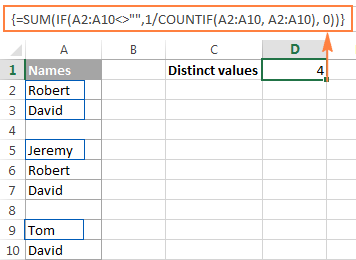#### Formula to count distinct text values

To count distinct text values in a column, we'll be using the same approach that we've just used to exclude empty cells.

As you can easily guess, we will simply embed the ISTEXT function into our Excel count distinct formula:

=SUM(IF(ISTEXT(range),1/COUNTIF(range, range),""))

And here's a real-life formula example:

`=SUM(IF(ISTEXT(A2:A10),1/COUNTIF(A2:A10, A2:A10),""))`

#### Formula to count distinct numbers

To count distinct numeric values (numbers, dates and times), use the ISNUMBER function:

=SUM(IF(ISNUMBER(range),1/COUNTIF(range, range),""))

For example, to count all different numbers in range A2:A10, use the following formula:

`=SUM(IF(ISNUMBER(A2:A10),1/COUNTIF(A2:A10, A2:A10),""))`

#### Count case-sensitive distinct values in Excel

Similarly to counting case-sensitive unique values, the easiest way to count case-sensitive distinct values is to add a helper column with the array formula that identifies unique values including first duplicate occurrences. The formula is basically the same as the one we used to count case-sensitive unique values, with one small change in a cell reference that makes a great difference:

`=IF(SUM((--EXACT(\$A\$2:\$A2,\$A2)))=1,"Distinct","")`

As you remember, all array formulas in Excel require pressing Ctrl + Shift + Enter.

After the above formula is finished, you can count "distinct" values with a usual COUNTIF formula like this:

`=COUNTIF(B2:B10, "distinct")`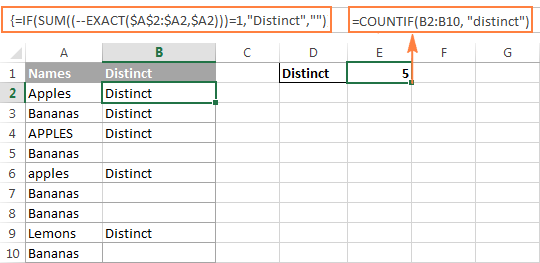If there is no way you can add a helper column to your worksheet, you can use the following complex array formula to count case-sensitive distinct values without creating an additional column:

`=SUM(IFERROR(1/IF(\$A\$2:\$A\$10<>"", FREQUENCY(IF(EXACT(\$A\$2:\$A\$10, TRANSPOSE(\$A\$2:\$A\$10)), MATCH(ROW(\$A\$2:\$A\$10), ROW(\$A\$2:\$A\$10)), ""), MATCH(ROW(\$A\$2:\$A\$10), ROW(\$A\$2:\$A\$10))), 0), 0))`

## Count unique and distinct rows in Excel

Counting unique / distinct rows in Excel is akin to counting unique and distinct values, with the only difference that you use the COUNTIFS function instead of COUNTIF, which lets you specify several columns to check for unique values.

For example, to count unique or distinct names based on the values in columns A (First Name) and B (Last Name), use one of the following formulas:

Formula to count unique rows:

`=SUM(IF(COUNTIFS(A2:A10,A2:A10, B2:B10,B2:B10)=1,1,0))`

Formula to count distinct rows:

`=SUM(1/COUNTIFS(A2:A10,A2:A10,B2:B10,B2:B10))`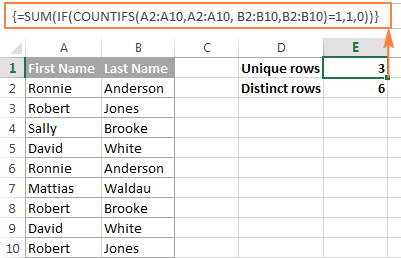Naturally, you are not limited to counting unique rows based only on two columns, the Excel COUNTIFS function can process up to 127 range/criteria pairs.

## Count distinct values in Excel using a PivotTable

The latest versions of Excel 2013 and Excel 2016 have a special feature that allows counting distinct values automatically in a pivot table. The following screenshot gives an idea of how the Excel Distinct Count looks like: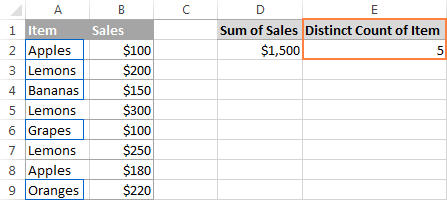To create a pivot table with the distinct count for a certain column, perform the following steps.

1. Select the data to be included in a pivot table, switch to the Insert tab, Tables group, and click the PivotTable button.
2. In the Create PivotTable dialog, choose whether to place your pivot table in a new or existing worksheet, and be sure to select the Add this data to the Data Model checkbox.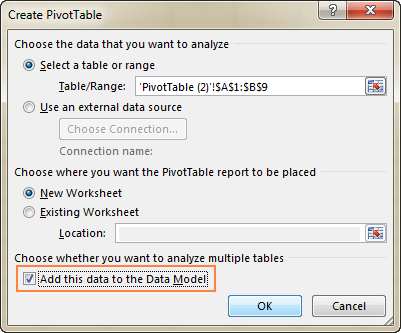3. When your pivot table opens, arrange the Rows, Columns and Values areas the way you want. If you don't have much experience with Excel pivot tables, the following detailed guidelines may prove helpful: Creating a PivotTable in Excel.
4. Move the field whose distinct count you want to calculate (Item field in this example) to the Values area, click on it, and select Field Value Settings… from the drop-down menu: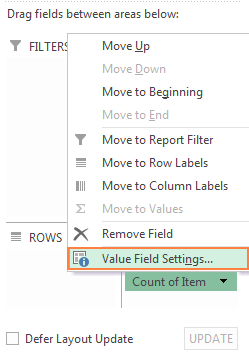5. The Value Field Settings dialog window will open, you scroll down to Distinct Count, which is the very last option in the list, select it and click OK.

You can also give a custom name to your Distinct Count if you want to.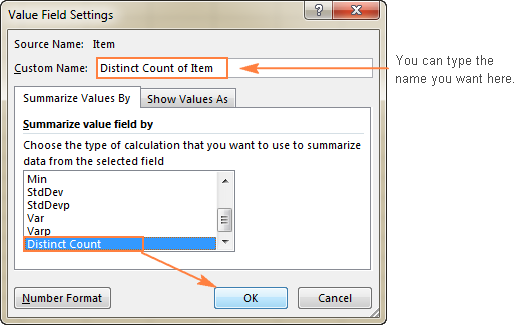Done! The newly created pivot table will display the distinct count like shown in the very first screenshot in this section.

Tip. After updating your source data, remember to update the PivotTable to bring the distinct count up to date. To refresh a pivot table, just click the Refresh button on the Analyze tab, in the Data group.

This is how you count distinct and unique values in Excel. If someone wants to have a closer look at the formulas discussed in this tutorial, you are welcome to download the sample Excel Count Unique workbook.

I thank you for reading and hope to see you again next week. In the next article, we are going to discuss various ways to find, filter, extract and highlights unique values in Excel. Please stay tuned!

### 78 Responses to "How to count distinct and unique values in Excel"

1.Fiona says:

Hi,
Would you please help me on this scenario. I have a spreadsheet where column a shows patient name. Column b shows type of service. Column a have duplicate patient names since some patient gets more than one services. Now I need to identify( count) how many patients were receiving more than one services. Would you help me with the fomular? Or a way to do that. Thank you very much.

•Svetlana Cheusheva (Ablebits.com Team) says:

Hello Fiona,

You can do it in the following way:

1. Add the following formula, say in column C, to identify duplicate names:
=IF(COUNTIF(\$A\$2:\$A2, \$A2)>1, "Duplicate", "")

Where A2 is the first cell in the Patient Names column.

2. Apply Excel's filter, filter out the unique name so that only duplicate names are visible, and copy those duplicate names to another sheet.

3. In that other sheet, use the following array formula to count distinct names (unique +1st duplicate occurrences). Remember to press Ctrl+Shift+Enter to complete the formula:
=SUM(1/COUNTIF(A2:A8,A2:A8))

Where A2 is the first and A8 is the last cell.

I've create a quick example for you, and you can download it here.

•John says:

After filtering out duplicates, what you have are unique names. So, applying CountA(A2:A8) would give the count of those names. Why do we need to apply the array formula as mentioned by you? Any specific reason?

•Svetlana Cheusheva (Ablebits.com Team) says:

Hi John,

Fiona's task was to count how many patients were receiving more than one services. For this, we needed to get a list of duplicates names, not unique names, and then count how many different (distinct) names appear in that list. I've re-worded point 2 in my previous comment, and hope now it makes more sense.

For example, after filtering out the unique names, we get the following list of duplicate names: Peter, Maria, Peter, Rob, Maria. And now, we need an array formula mentioned above to count how many different names appear in this list (3 names). You can find the detailed explanation of the formula in How the distinct formula works.

2.Manikandan C says:

Dear all,

i need to unq name wise sum of the Amount. not pivot required. Only formula.

Ex:-
Name Amount
A 391
B 145
C 268
D 514
A 526
C 991
B 456

Ans should be:-

Count Amount
4 1318

3.akshay says:

hi,
How to count the number of distinct values. If the values are neither numbers nor text but the mix of both like 12000078fg?

4.Dani says:

Hello,

We download a report from Just Giving each time we have a donation and the format is not very easy to follow so we would just like to extract the information we need (eventually in a macro).

I would like to lookup all the distinct values in the user ID column, then calculate the total number of donations for each of the distinct ID values. I can then apply this formula to the other fee columns.

Any help would be much appreciated!

Many thanks,

Dani

5.Fab says:

Hi,

I don't see the item "Add this data to the data model" in the Create Pivot Table window. How come? I am using Excel 2010.

•Svetlana Cheusheva (Ablebits.com Team) says:

Hi Fab,

Regrettably, this option is not available in Excel 2010. It was introduced in Excel 2013, and also exists in Excel 2016. In earlier Excel versions, you can count unique values using formulas as demonstrated in the first parts of this tutorial.

6.Derek says:

I have Excel 2013, but the checkbox for "add this data to data model" is grayed-out and unchecked. Is there an Excel Add-in required to get this area functional?

Thanks,
Derek

7.Ivan says:

Hi,
In Excel 2013 I have a table with more fields. The table is sorted on first field. In a new generated field of my table I have to count for each row the number of rows in the table depending on filter of the sorted field and also depending on filters of few other fields. I could solve this problem with COUNTIFS function, but there is another condition. One of the "fileds - filter" must be distinct, the others may be duplicates too.

?

Any help would be much appreciated!

Many thanks,

Ivan

8.Anand Kumar says:

Nice Article.........

Thanks ☻.

9.Ranjeet Ranjan says:

I did not find option Add data modeling during creating pivot table in Excel 2016

10.megann mcdaniel says:

I have Excel 2013 and use Pivot tables often. I tried using the Count Distinct Values feature but it is not listed in the Value Field Settings. Is it possible I need to install an update?

11.Shruthi says:

Hi I want the formula to count how many clients have completed by one person according to the date.
Client Name Auditor name Date
SFM Realty Corp. Shruthi 10/25/2016
SFM Realty Corp. Shruthi 10/25/2016
SFM Realty Corp. Shruthi 10/25/2016
American Kiosk Shruthi 10/26/2016
American Kiosk Shruthi 10/26/2016
American Kiosk Shruthi 10/26/2016

Here I need the Count for the Auitor name Shruthi Audited clients as 1 on 10/25/2016 & 1 on 10/26/2016.It should take the distinct names in the clients coloumn.

12.Chetan says:

Hi I want to count values excluding the duplicates.
For example, I have two materials with different batch numbers for each material which contains 10 containers each belonging to multiple shipping lines. from this 20 containers of two batches, I want to count no. of containers under each shipping lines by using countifs and criterias are material name, batch number and shipping line and type of container (20' or 40'). Each containers weighs 24.75 tons but I have one container 12.375 in first batch and 12.375 in another batch. I don't want this container to be counted twice when I enter the batch number.

13.agostonz says:

Hi, I wish to all af you nice & new challanges, for 2017.
I have my own and asking for your help... My data are as below, in Excel 2013:
-column A: incomming invoice date (can be more identical, as there are more invoices on the same day). Like jan01, jan01, jan02, jan15, jan15, ...
The column is filtered, / Month or whatever.
I need to count the dates where there is only one input (row) in the filtered range (needed result is 1 (jan02) from above example, as that is the day when just one invoise was received.
There is no option to add more columns. Is it possible to get the right result by using the date column only?

14.LW says:

How would I count distinct dates in a selection of cells? I tried changing the dates to number format and then using =SUM(IF(ISNUMBER(range),1/COUNTIF(range, range),"")), but I got an incorrect answer with an error saying that the formula "omits adjacent cells".

15.christy says:

Hi,

I forgot to select Add this data to the Data Model checkbox.what to do now

16.Paul says:

First - thank you so much for this site. Very helpful.

I have a spreadsheet with a large number of distinct account numbers. Each time an account number appears on the spreadsheet, whether duplicate or unique, it represents a different transaction with a value for each transaction. There are 3 columns, (A) for acct. #; (B) for transaction 1; and (C) for interest on transaction 1. I would like to get a list of distinct account numbers with the sum of the values for each distinct acct. number. (At the end, the list would contain a column for: (A) Distinct Acct.#; and, (B) sum or total transaction value for all values for each acct#; and (C) total or sum of interest for each acct#.

•Irina Pozniakova (Ablebits.com Team) says:

Hello Paul,

Thank you very much for your feedback, we're happy to hear you find our site helpful.

It sounds like the Subtotal option in Excel will do what you need:
- Select your records and go to Data tab
- Click on Subtotal and choose the following options:
- At each change in - column with the account number, use function - SUM, add subtotal to - select the columns with the values you want to sum.
- Click OK

I hope this helps.

•Paul says:

Yes - absolutely worked. Wonderful. Thank you!!

17.Adarsh says:

my inputs are

Abc 2 500
def 1 2000
abc 4 300

Output should be:
Abc 6 800
def 1 2000

18.Ashok says:

Hi Team,

Need help in adding total development hours of particular developer,irrespective of their name in Dev1, Dev2 or Dev3

Ticket ID Dev1 Dev2 Dev3 #Dev1hrs #Dev2hrs #Dev3hrs
1 Basanth Sriram Shoks 10 12 10
2 Shoks Basanth Sriram 5 10 17

19.Tugcan says:

Hi,

I have an excel sheet for training records which has columns named "name of course", "instructor", "course number", "attendee", "duration"etc. As I have multiple attendees on each training, training number is remaining same for different attendees. I would like to see the total duration for each instructor seperately on pivot table however, when I use "sum of duration" as a pivot table column, it is calculating all the numbers shown under duration column. (I mean, if there are 5 attendees for same training and if the duration is 8 hours, value that I want to see on pivot table is 8 hours, unfortunately it multiplies the number of attendees and duration and seems 40 hours) Is there a way to solve this problem?

20.Rodrigo says:

Thank you! It helped a lot!

21.Donna says:

I have pulled data from several states with account, I want to look at only one state & only count unique Business in that state, no duplicates

22.Greg says:

Hello
I am a teacher trying to use google sheets to gather data. I'd like to break each assessment question into two parts: one for understanding the concept, and the other for computation. The only value that will go in each will be an x indicating that there was an error. I then want to accumulate separate totals based on these entries. Because this same rule doesn't apply to every question, not all questions will have a concept and computation part.

I am trying to use COUNTIF as follows:
COUNTIF(C7,"x")+(G7,"x")+(I7,"x") etc. I am getting a Formula parse error in the cell.

•Natalia Sharashova (Ablebits.com Team) says:

Hello, Greg,

I'm sorry, but it's not exactly clear what result you want to get. Maybe this formula will do:
=COUNTIF(C7,"x")+COUNTIF(G7,"x")+COUNTIF(I7,"x")
If you still need our help, you can send us a small sample workbook with your data and the result you want to get to support@ablebits.com. Don't forget to mention this article and your comment.

23.George says:

you guys r awesome

24.Chuck says:

Hello there,

May I ask, is it possible to exclude blanks when using Distinct Count in a pivot table?

Thanks in advance for any guidance! :)

Chuck

25.Peter says:

Great page!
However, when I copy/paste the formula and enter the correct ranges, I get an error message, not recognising this as a valid formula.
I have had this issue with other formulas too, so it might be a cell formatting issue?

26.Ted T says:

Peter, this is a Array Formula. To activate you must select CTRL+SHIFT+ENTER to activate. This will automatically add the { and } characters to the formula. They can not be added manually.

Hope this helps!

Many thanks to the folks at AbleBits for this tutorial and examples. I could not get my counts to work correctly.

On a side note, I've been burned by bad data in the past so I also recommend trimming your data to remove any trailing spaces and removing any punctuations like the ' in O'Brien. If your data contains mixed case I would also recommend reformatting everything to the same case format. O'Brien and Obrien both become OBRIEN. You'll be happy you did.

27.Tina says:

Hi
Excellent page and tutorial!
I have a pivot table created with patients coming in every month. I want to count the new patients that have come in each month, so basically compare the previous months data and put as null if the patient visited the previous months.

28.Rahul says:

Hello! I need help
I have a list and I want to count the product in this way.

product A = 1
product A = 1
product A = 1
product B = 2
product B = 2
product C = 3
product D = 4
product D = 4
product D = 4
product D = 4

•Gennady Terekhov (Ablebits.com Team) says:

Hello, Rahul,
For me to understand the problem better, please send me a small sample workbook with your source data and the result you expect to get to support@ablebits.com. Please don't worry if you have confidential information there, we never disclose the data we get from our customers and delete it as soon as the problem is resolved.

29.BERNAL says:

Hi,

I have sought to use the following formula:

=SUM(IF(COUNTIF(A2:A10,A2:A10)=1,1,0))

No matter how implemented it returns 0. I have even created some simple new text data in a new column (Jim, Bob, Jim, Sally, Joe, Bob, Sally, Joe, Jim) in a new test spreadsheet, and the same happens.

I can only conjecture that the reason for this is that COUNTIF(RNG1,RNG1) returns an array, not a single number, and so produces 0?

The concept seems so simple, but I have spent that last few hours trying to resolve this maddening issue.

B

•Gennady Terekhov (Ablebits.com Team) says:

Hello,

This is an array formula, so be sure to press Ctrl + Shift + Enter to complete it properly. Once you do this, Excel will automatically enclose the formula in {curly braces}. Typing the curly braces manually won't work.

30.Victor says:

Hi Guys,

How would you ensure that duplicates are not included for the first criteria i.e ('GRP310 for P&L Variance'!\$C\$5:\$C\$1000='P&L Variance MTD'!B\$5) ???

=+SUMPRODUCT('GRP310 for P&L Variance'!\$E\$5:\$BZ\$1000,('GRP310 for P&L Variance'!\$C\$5:\$C\$1000='P&L Variance MTD'!B\$5)*('GRP310 for P&L Variance'!\$D\$5:\$D\$1000='P&L Variance MTD'!\$A7)*('GRP310 for P&L Variance'!\$E\$3:\$BZ\$3='P&L Variance MTD'!B\$6)*('GRP310 for P&L Variance'!\$E\$4:\$BZ\$4='P&L Variance MTD'!\$A\$5))

•Gennady Terekhov (Ablebits.com Team) says:

Hello Victor,
For me to understand the problem better, please send me a small sample workbook with your source data and the result you expect to get to support@ablebits.com. Please don't worry if you have confidential information there, we never disclose the data we get from our customers and delete it as soon as the problem is resolved.

31.prakash says:

how to count each sales person bill number and unique

32.Husnain says:

Dear, I am working on the attached Sheet. I need to calculate the Values in Column "G" i.e. to Count unique text values based on multiple (Two) criteria, but criteria are in NUMBERS not in TEXT. Further, Its is big sheet, therefore I want to use the cell reference in range and in criteria. I am very confused. I want to count the column C i.e. Degree based on the Criteria E and F. That is, Look E2 in column A and Look F2 in column B and count the unique text values in Column C. Hope it is clear.
Plz help. Thank you

•Gennady Terekhov (Ablebits.com Team) says:

Hello,
For me to understand the problem better, please send me a small sample workbook with your source data and the result you expect to get to support@ablebits.com. Please don't worry if you have confidential information there, we never disclose the data we get from our customers and delete it as soon as the problem is resolved.

33.Coco says:

Hi,

I'm working with Excel 2010. I'm working on a document that keeps track of all our projects. I need to know how many organizations we worked with in a month period. We keep track of every interaction so the same organization appears multiple times in the list. How can I have the number of distinct organizations by a date range?

I use this formula to get all the organizations in the column, but I can't figure out how to condition it by date range

=SUMPRODUCT((' Activities'!K2:K100"")/COUNTIF(' Activities'!K2:K100,' Activities'!K2:K100&""))

Any help is very much appreciated.

Thank you

34.Reggie says:

Hello,

Similar to Fiona's question posted 09 April 2016, I have a list of patients in column b with duplicates, their procedure in column c, and the date of the procedure in column d. I need to report how many patients received each kind of procedure quarterly. Is it possible to have a formula that will report this information in the same worksheet? I have been able to figure out how to count how many "unique" patients for the whole year, but not in a date range based on each procedure.

Thanks in advance for any help.

35.Pandu says:

Hi Svetlana Cheusheva,
I want to count no of billing documents with Order number wise and number of billing dates for order number.

Order number Billing Date No of billing Dates Billing Document No of invoices
2407571 18-Jan-18 1 8014769109 1
2407573 8-Jan-18 1 8014769017 1
2407574 8-Jan-18 1 8014769017 1
2407575 5-Jan-18 3 8014769004 3
10-Jan-18 8014769041
16-Jan-18 8014769086
2407576 16-Jan-18 1 8014769086 1
2407577 9-Jan-18 8014769018
2407578 5-Jan-18 8014769002
9-Jan-18 8014769025
16-Jan-18 8014769083

•Ekaterina Bespalaya (Ablebits.com Team) says:

Hi Pandu,

I am sorry, your data look distorted in the comment above. If you can send us a small sample workbook with your data and the result you want to get, we'll be able to help you better.
Please shorten your table to 10-20 rows / columns and email it to support@ablebits.com. Please also don't forget to include the link to your comment in the email.

Thank you.

36.José says:

Hello,
I'm using Excel 2016, and used Distinct Count within a pivot table.
My result looks like this:
Row Label Distinct Count
Item 1 1
Item 2 39
Item 3 3
Grand Total 40
See, the grand total does not match the sum of each item. I've checked each item's details and I figure the grand total should be 43 (1+39+3) instead of 40 that the pivot calculates.
Any ideas on why this might be different?

Thank you.

37.Karolis says:

Hello,

I need to count the number of distinct text and number values in column \$M:\$M , when in column \$E:\$E is written "*italija*", and when in column \$F:\$F is written "saus", also - it should not count blank cells.

Now I am doing this in pivot: filtering column F with "saus", than filtering column E with "italija", than copying column M in another sheet, removing dublicates and counting the cells.

•Ekaterina Bespalaya (Ablebits.com Team) says:

Hello, Karolis,

Would it be possible for you to send us a small sample workbook with your source data and the result you want to get? Please shorten your table to 10-20 rows / columns and email it to support@ablebits.com. Please also don't forget to include the link to your comment in the email.

38.Brad says:

For "distinct" data, ie, I needed to know how many individual customers I had in a list of transaction I did this:
Sorted data by customer name, then added
a column of the formula: =IF(EXACT(A2,A3)=TRUE,0,1) in each row,
then summed the column.
Easy, accurate, no special arrays etc. had to hide the column when sharing.

39.Donna says:

I created a pivot chart using distinct values and now want to group the dates portion of the data to get distinct counts by month, however, the group function is grayed out. How can I group this information if the group function is not available without manual working / adding columns? Thank you.

40.SV says:

41.Sian says:

Hi,

Thank you for all of the information on here.

I am experiencing a range limit on the use of this calculation that is much lower than 125. For some reason it is only letting me check 20 rows, anything above that is returning a result of 0. Is this normal? Is there anyway around it.

What I really want to do is check an entire column in a table for values (there is about 1000), but as soon as i do this the returned value is zero. If it is less than 20, the returned value is acurate.

I am using;
=SUM(IF(ISTEXT(A1:A20)*COUNTIF(A1:A20,A1:A20)=1,1,0)) - works

=SUM(IF(ISTEXT(A1:A30)*COUNTIF(A1:A30,A1:A30)=1,1,0)) - total is zero

42.Rah says:

Hi,

I have a survey with hundreds respondents which the answers are their hometown. For example:
London
Milan
Basel
Tokyo
Paris
Tokyo
Paris
Basel
Tokyo
Amsterdam
Basel
Amsterdam
Amsterdam
Amsterdam

I want to count how many people come from each city. The result I want is like this
Amsterdam 4
Basel 3
Tokyo 3
Milan 1
London 1
Paris 2

Is there any solutions to do it automatically?

43.Javier Castorena says:

I need to count unique values based on:
- unique values storage (SAP sheet column B)
- on a specific date (SAP sheet column C)
- on a production line (SAP sheet column G).

I need to storage the data in sheet DataBase:
- Column W for produciton line 3 based on date on column I
- Column 0 for producion line 4 based on date on column I

I sent you the file to support@ablebits.com (file called T1XX- from Javier Castorena)

44.Rajan says:

I want to know that How can I get no. of unique company with user wise with formula in excel.

Example:-

Commapny Name User

WeTalkive Jalpesh
WeTalkive Jalpesh
Codeveloped BV Brijesh
Codeveloped BV Brijesh
The Red Corner B.V Jalpesh
The Red Corner B.V Jalpesh
Jumbo Golf Brijesh
Jumbo Golf Brijesh
Jumbo Golf Brijesh
Jumbo Golf Brijesh
JEKA Industriële Efficiency Jalpesh
JEKA Industriële Efficiency Jalpesh

45.Jaime says:

How to use subtotal to make counting filter dependent?

46.David G says:

I am attempting to count a column of 7-9 digit (patient medical record) numbers, excluding any duplicates and only if a specific value (ie: MICU) is listed in another field of the same row. (Basically I need to count how many different patients where in a specific unit.) Can you help? I have only gotten as far as counting unique patient numbers.

47.Azhar Husain says:

Hello There,

I have data of my sales and which is having SKU ID which is not in same form and i want to count with COUNTIF formula however i am not able to do that, kindly advise.

48.Des says:

How to count total quantity per unique parts and identify fast moving items. Pls help
Example
Screw 7 amount 80usd
Hose 3 60usd
Screw 10 amount 80usd
Keyboard 5 5usd
Hose 5 60 usd
Thanks a lot

49.Katie says:

I need to count the number of distinct text values in a column (ignoring blanks) but the following formula is returning an incorrect value of "1".

=SUM(IF(ISTEXT(range),1/COUNTIF(range, range),""))

50.Donis says:

Hello,
I want to find exact text located in two cells in one column and cout it. How to do this?

For example:
Need to find how many times 'cat black' is repeated in one column:

Cat
Blue

Cat
Black

Cat
White

Cat
Black

And so on

In the example the count of 'cat black' must be 2.

Thanks for help.

51.Victor says:

Hi,
How can I count unique value within a range IF these values match those of another column? For example, in column A I have Apples, Oranges, Bananas.
In column B I have several types of cars.
How can I count the different types of car associated with each fruit (eg. banana)?

52.Jayendra Koirala says:

=IF(ISNUMBER(MATCH("Apples",M:M,0)),COUNTIF(N:N,"Green"),0)
This formula is not perfect. I want to see if there are "Apples" in one column and "Green" in corresponding column, it should count all Green Apples.
Thanks.

53.Samuel Dighan says:

None of this works. Rubbish article.

•Svetlana Cheusheva (Ablebits.com Team) says:

Hello Samuel,
You can download our sample workbook and make sure all the formulas work exactly as described.

54.Jinky Zuniga says:

Hello, Im Trying to create a formula for this one, I need to count distinct values per week, only the “Open” status. The hard part is, you dont need to count the”Open” status if at a certain time that week it was already closed.

SR Reference# Week # Status
19999 32 Closed
189898 32 Open
189898 32 Closed
189898 29 Closed
189898 31 Open
19999 33 Open
19999 33 Open

55.Henry says:

A B
00:00 E/F
01:00 E/F
02:00 E/F
03:00 10
04:00 E/F
05:00 E/F
06:00 5
07:00 10
08:00 L/S
09:00 L/S
10:00 L/S
11:00 L/S
12:00 L/S
13:00 L/S
14:00 12
15:00 P/O
16:00 P/O
17:00 P/O
18:00 P/O
19:00 O/C
20:00 O/C
21:00 O/C
22:00 E/F
23:00 E/F
There are 3 sets of E/F here.How will i get excel to output 3 counts? That is 1 count for each set of E/F.Thanks

56.Ramki says:

Month Agent Sales Agent Status
JAN A 0.02 A 1
JAN B 0.00 B 1
JAN C 0.02 C 1
JAN D 0.03 D 1
JAN E 0.06 E 1
JAN F 0.05 F 1
FEB A 0.00
FEB B 0.00
FEB C 0.04
FEB D 0.02
FEB E 0.01
FEB F 0.00
MAR A 0.04
MAR B 0.03
MAR C 0.02
MAR D 0.06
MAR E 0.05
MAR F 0.06
Hi...
Request you to please go thru' the above concern.
We have 6 agents (A,B,C,D,E,F) did sales more than 2k (it's a must) a period of 3 months.
At the end of quarter we wish to know all agents did one time sales i.e.>=2k (either one time or more) or not.
If we have the data like above, please suggest a formula.

57.Fares Odeh says:

Dear All,
Please i need help, I want to count unique values with criteria and reported to a general summery to another "sheet".

58.Rishabh Jain says:

Dear all,

Could you please elaborate the working of this formula: "=SUM(IFERROR(1/IF(\$A\$2:\$A\$10"", FREQUENCY(IF(EXACT(\$A\$2:\$A\$10, TRANSPOSE(\$A\$2:\$A\$10)), MATCH(ROW(\$A\$2:\$A\$10), ROW(\$A\$2:\$A\$10)), ""), MATCH(ROW(\$A\$2:\$A\$10), ROW(\$A\$2:\$A\$10))), 0), 0))".

59.Rishabh Jain says:

Dear all,
Request you to kindly help me understand this formula:
=SUM(IFERROR(1/IF(\$A\$2:\$A\$10"", FREQUENCY(IF(EXACT(\$A\$2:\$A\$10, TRANSPOSE(\$A\$2:\$A\$10)), MATCH(ROW(\$A\$2:\$A\$10), ROW(\$A\$2:\$A\$10)), ""), MATCH(ROW(\$A\$2:\$A\$10), ROW(\$A\$2:\$A\$10))), 0), 0)). I posted a comment earlier as well and it was removed.
Thank you!

60.MURALY says:

AN EXCEL SHEET CONTAINS DATA
EG:-IN CERTAIN CELLS CONTAIN ONLY A NAME (Eg:- "RONY")
IN CERTAIN CELLS CONTAIN TWO or more NAMES (Eg:- "RONY,SONA")
HOW CAN I COUNT ONLY ONE PARTICULAR NAME IN THAT SHEET using formula?

61.Willem says:

I have two columns. Column A contains 5 different treatments for vehicles, let's say treatments S, T, U, V and W. These five treatments are applied to vehicles in column B. The same vehicle might receive more than one treatment per month, sometimes the same treatment twice. I want to know how many distinct vehicles in column B received treatments S, T and V. Thus distinct values based on multiple OR criteria from the same column A. Typical 2500 to 5000 rows per month.

62.Shiran jayathilaka says:

You can use sumif function.
Before that, pls insert new coloumn and enter "1" in to all lines.

And select and copy all names in to new coloumn.and remove the duplicates.and use the sumif function and get the sum of services each patient.

63.Chresl Baylis says:

How do you get this formaula to also ignore errors as well as Error values such as "#NUM"

64.David Porte says:

Hello, I apologize in advance for asking for help but hopefully, this is an easier one for you, less so for me.
I have a large data set with one unique identifier, that being the VIN number of a vehicle. When we process a service transaction for a vehicle, sometimes there will be more than one tech working on the same vehicle and that will require more than one entry into the spreadsheet. To accommodate this requirement I have set up a macro that copies all of the data on the selected row and places a duplicate on the next available row. The problem I have is that in doing so, it creates a duplicate of everything including several items that I don't want to be duplicated such as total job hours and total hours quoted as these will distort the records if my staff fail to remove these manually each time.

What I am trying to achieve is to have a formula that can be used in the total hour's sum cell (B50) that detects the duplicated record created by the macro having detected the VIN as identical to the same VIN already used previously in that column, A1:A50.

The problem I am trying to solve occurs because the entire job hours and job \$ values are recorded as a whole when the record is first created. The macro then creates an entirely new duplicate record though artificially doubling the sum value by adding new row with a clone of the same data. I want to keep the clone data as-is but I just don't want to count the additional hours that now appear in the hour's column C1:C50.
I intend to use the same formula for several items including hours and all dollar values for that job as the problem affects several cells. I just need one working formula and I can modify it from there for each column.
I sincerely hope that makes sense.I am happy to send you the sheet if that makes this easiler to understand.

60+ professional tools for Excel